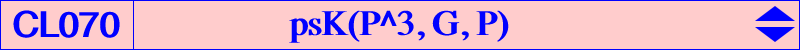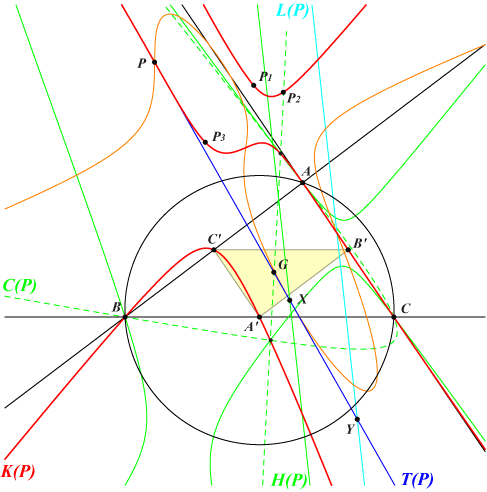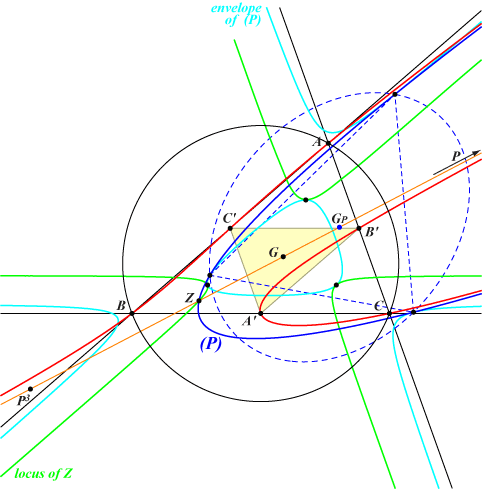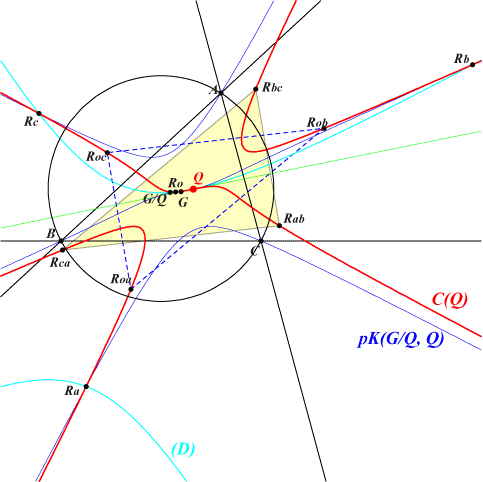Let P = p : q : r be a point and denote by K(P) the cubic psK(P^3, G, P) where G is the centroid of ABC. The equation of K(P) is : (q - r)(r - p)(p - q) x y z + ∑ p^3 (y -z) y z = 0. When P lies on a sideline or on a median of ABC, K(P) splits into this same line and a conic. In particular, K(G) is the union of the medians of ABC. These special cases are excluded in the sequel. Properties of K(P) • K(P) is a circum-cubic of ABC and the tangents at A, B, C concur at P^3. • K(P) is a circum-cubic of the medial triangle A'B'C'. • K(P) passes through P which is a point of inflexion. The inflexional tangent T(P) passes through G and the polar conic of P splits into T(P) and the harmonic polar H(P) of P. H(P) is the image of the trilinear polar L(P) of P under the homothety h(P, 2/3). T(P) and H(P) meet at X, the center of the polar conic of P, hence X (and P) must lie on the (orange) Hessian of K(P). • K(P) is globally invariant under two transformations 𝛗1 and 𝛗2 which are inverse of one another : for any point M on K(P), M1 = 𝛗1 (M) and M2 = 𝛗2 (M) are also two points on K(P). M1 is the G-Ceva conjugate of the P^3 isoconjugate of M and M2 is the P^3 isoconjugate of the G-Ceva conjugate of M. With M = x : y : z, this gives : M1 = p^3 y z (-p^3 y z + q^3 x z + r^3 x y) : : and M2 = p^3 y z (x-y+z) (x+y-z) : : . In particular, K(P) passes through P1 = 𝛗1 (P) = p^2 (-p^2+q^2+r^2) : : and P2 = 𝛗2 (P) = p^2 (p+q-r) (p-q+r) : : , on a line passing through P. • P3 = 𝛗1 (P1) and P4 = 𝛗2 (P2) are the respective tangentials of P2 and P1, also on a line passing through P.• K(P) meets the line at infinity at the same points as pK(G/P, aP2) where G/P is the G-Ceva conjugate of P and aP2 the anticomplement of P2. These two cubics meet again at six finite points of a same conic C(P) namely A, B, C, P4 and two points on the line GP2.   L(P) and T(P) meet at Y. X is h(P, 2/3)(Y), on the (orange) Hessian of K(P). Recall that X is the center of the (decomposed) polar conic of P.K(P) with P on the line at infinity When P is an infinite point, K(P) becomes a trident and its pseudo-pole P^3 lies on K656, the image of K015 = cK(#X2, X2) under h(G, 3/2). K(P) has the line GP as an asymptote but it has now a parabolic asymptote (P) meeting GP at the image Z of P^3 under h(G, 1/3). It follows that Z lies on the image of K015 under h(G, 1/2). See green curve below. Apart the points A, B, C, A', B', C', P mentioned in the general case, K(P) now also passes through P^4 and obviously its successive images under 𝛗1 and 𝛗2.When P traverses the line at infinity, (P) envelopes a cubic which is the image under h(G, 1/2) of the Tucker cubic obtained when 𝝺 = – 4/11. See light blue curve. (P) is tritangent to this cubic and the centroid GP of the triangle of contacts lies on GP and on the image of K015 under h(G, – 1/3). *** See K1152 = K(X523) where further properties are given.A generalization If M is an infinite point, the cubic psK(Ω, P, M) is a trident if and only if : • the pseudo-pole Ω lies on pK(M^4, M) passing through M, M^2, M^3, • the pseudo-pivot P lies on pK(G, tM) passing through G, M and the isotomic conjugate tM of M. This gives the cubics psK(M, tM, M) of CL063 and psK(M^3, G, M) of CL070. psK(M^2, M, M) = pK(M^2, M) is not a proper trident since it is the union of three parallels passing through A, B, C.Some examples Peter Moses has computed a selection of cubics K(P) passing through at least three ETC centers. Those in the first table are tridents.P on L∞ X(i) on K(P) for i = 30 30, 9410, 14993, 36435 512 512, 9428, 9494 513 513, 798, 9296, 36957 514 514, 1577, 3669, 6631, 36954 518 518, 9470, 33675 519 519, 9460, 36909 522 522, 4130, 10001, 24006, 36956 523 523, 2489, 23285, 31998, 36953

With P on the line at infinity L∞, K(P) contains the points P^4, 𝛗1 (P^4) and 𝛗2 (P^4).

𝛗1 (P^4) is the G-Ceva conjugate of the isotomic conjugate tP of P. It is the center of the circum-conic with perspector tP and vice-versa. See red points.

𝛗2 (P^4) is the perspector of the bicevian conic C(G, tP). See green points.

The only listed P^4 is X(36435) = X(30)^2. See blue point.

See K1152 = K(X523.P not on L∞ X(i) on K(P) for i = 1 1, 3, 56, 36103 3 3, 1092, 1147 4 4, 6523, 6526 6 6, 206, 1974 8 8, 6552, 6556 9 9, 480, 6600 10 10, 1089, 4075 57 57, 6609, 6612 63 63, 6503, 6512 75 75, 6374, 6383 487 487, 6337, 33365 488 488, 6337, 33364 650 650, 8641, 17115 30556 220, 1124, 30556 30557 220, 1335, 30557

If Q is a finite point different from G, the locus of P such that K(P) passes through Q is a central cubic C(Q) with center Q where the inflexional tangent passes through G. With Q = p : q :r , the equation of C(Q) is :

p q r (y - z) (z - x) (x - y) + ∑q r (q - r) x^3 = 0.

For instance, C(X3) is K100 passing through X(i) for i in {1, 3, 40, 1670, 1671, 17749}.

C(Q) meets the line at infinity at the same points as pK(G/Q, Q) where G/Q is he G-Ceva conjugate of Q. The six other (finite) common points are Q (counted twice) and the four (real or not) square roots Ro, Ra, Rb, Rc of G/Q.

These latter points lie on the polar conic (D) of Q in pK(G/Q, Q)which is a diagonal conic in ABC. (D) also contains G/Q, the vertices of the anticevian triangles of Q and G/Q, the (real or not) square roots of every point on the line passing through Q and G/Q. The equation of (D) is :

∑q r (q - r) x^2 = 0.

C(Q) obviously passes through the reflections of Ro, Ra, Rb, Rc in Q.C(Q) also passes through the six midpoints of every pair of points in {Ro, Ra, Rb, Rc}, the midpoint of RxRy being denoted by Rxy. These midpoints are two by two symmetric about Q and lie on the circum-conic of ABC with center Q and perspector G/Q. *** The figure opposite is made with Q = X(1) hence G/Q = X(9) and pK(G/Q, Q) is K157. Ro = X(188) and (D) is the diagonal rectangular hyperbola passing through X(i) for i = 1, 9, 40, 188, 191, 366, 1045, 1050, 1490, 2136, 2949, 2950, 2951, 3174, etc. More generally, (D) is a diagonal rectangular hyperbola for every Q on the Thomson cubic K002 and then, the line Q, G/Q passes through the Lemoine point X(6), the isopivot of K002. In this case, the circum-conic passing through Q and G/Q is also a rectangular hyperbola and the center of (D) lies on the circumcircle of ABC.Note that the center of (D) lie on the circum-conic of ABC with center S (and perspector G/S) if and only if Q lies on pK(G/S, G). With S = X(3) hence G/S = X(6), we find the Thomson cubic as above.